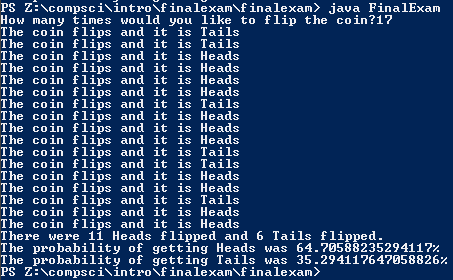# Final Exam

## Code

```    /// Name: Kabir Virk
/// Period: 6
/// Program Name: Final Exam
/// File Name: FinalExam.java
/// Date Finished: 1/22/2016

import java.util.Random;
import java.util.Scanner;

public class FinalExam
{
public static void main( String[] args )
{
Scanner keyboard = new Scanner(System.in);
Random rng = new Random();

int Another = 0;

Tails = 0;
//numbers start at 0
System.out.print("How many times would you like to flip the coin?" ); //asking the question
flips = keyboard.nextInt();

while ( flips < 1 || flips > 2100000000 )
{
System.out.println ("Sorry that number is ");

if ( flips < 1 )
{
System.out.println ("too small");
}
else if ( flips > 2100000000 )
{
System.out.println ("too large");
}

System.out.println("Please pick a number between 1 and 2,100,000,000. ");
flips = keyboard.nextInt();
}

do
{
int flip = rng.nextInt(2);
String coin;

if ( flip == 1 )
{
Another++;
}
else
{
coin = "Tails";
Another++;
Tails++;
}
System.out.println ("The coin flips and it is " + coin );
} while (Another < (flips) );
//Using while loops to repeat coin flips
double probabilityoftails = (double)Tails / flips;

double percenttails = (probabilityoftails * 100);

System.out.println ("There were " + Heads + " Heads flipped and " + Tails + " Tails flipped."); //tells number of heads and tails
System.out.println ("The probability of getting Tails was " + percenttails + "%");//tells percent of tail

//after running the program, I found that the numbers that give percentages closest to 50% are numbers that end in 0 because it is a multiple of 10, this is because you multiply it by 100 to find the percent
}
}

```

### Picture of the output